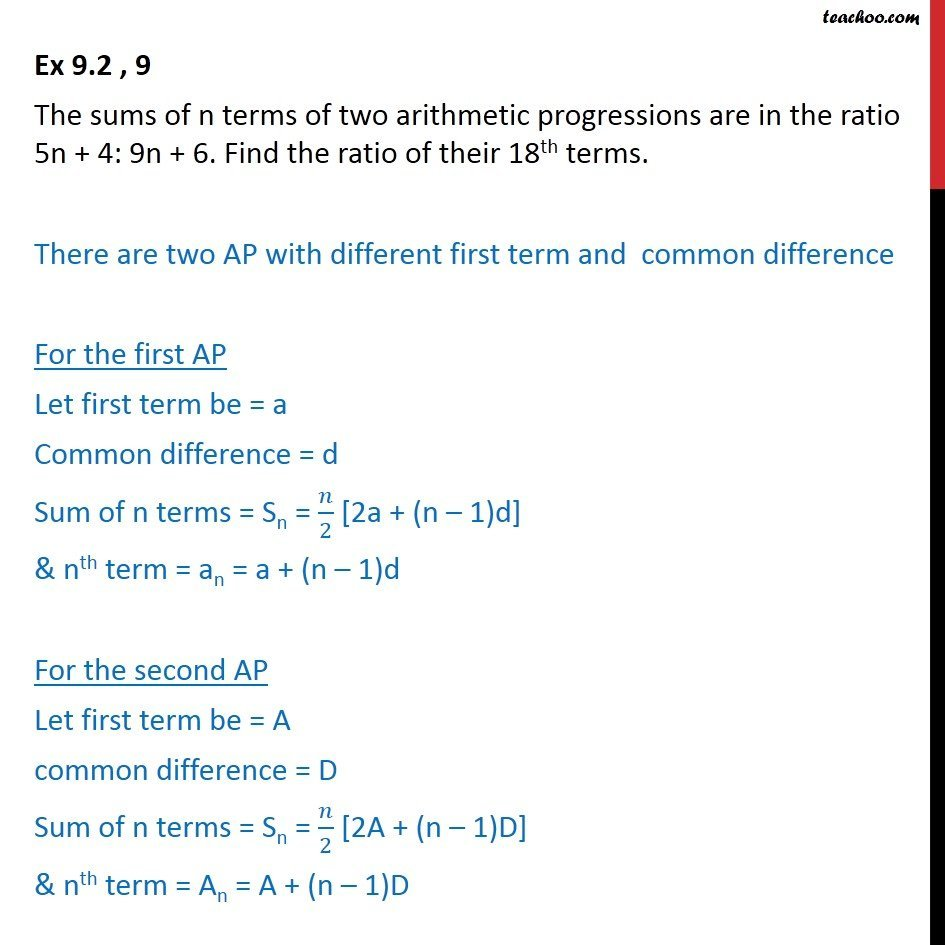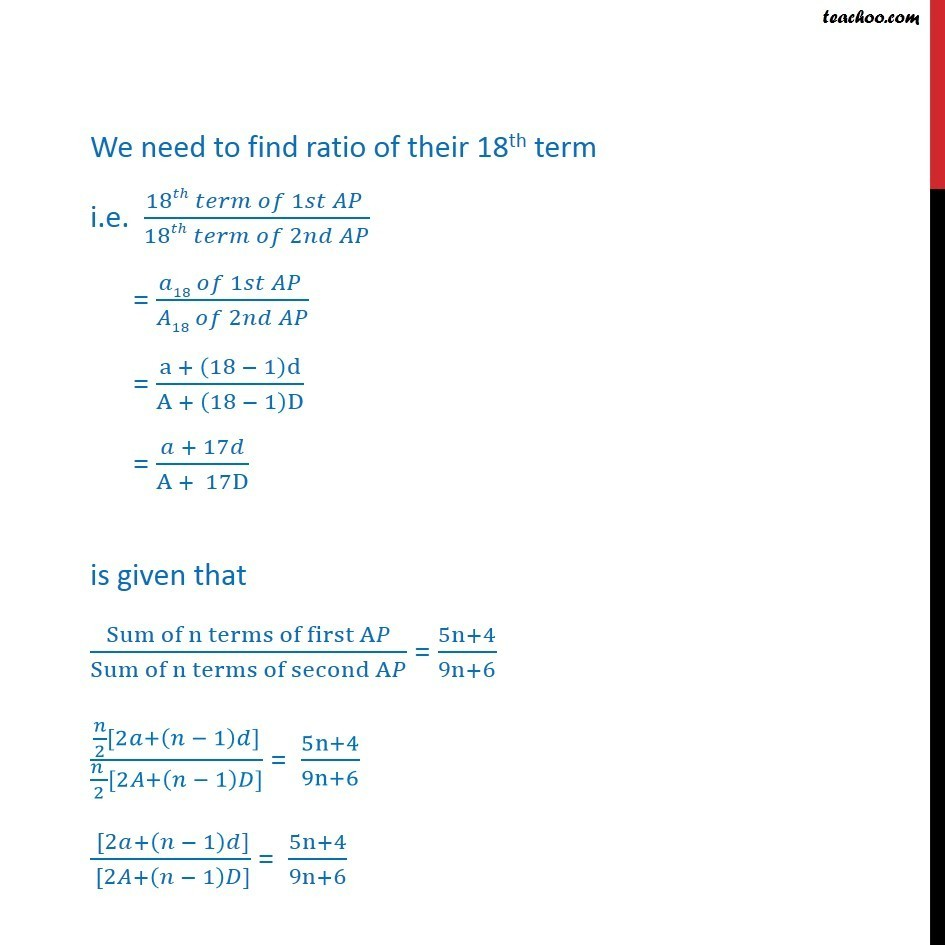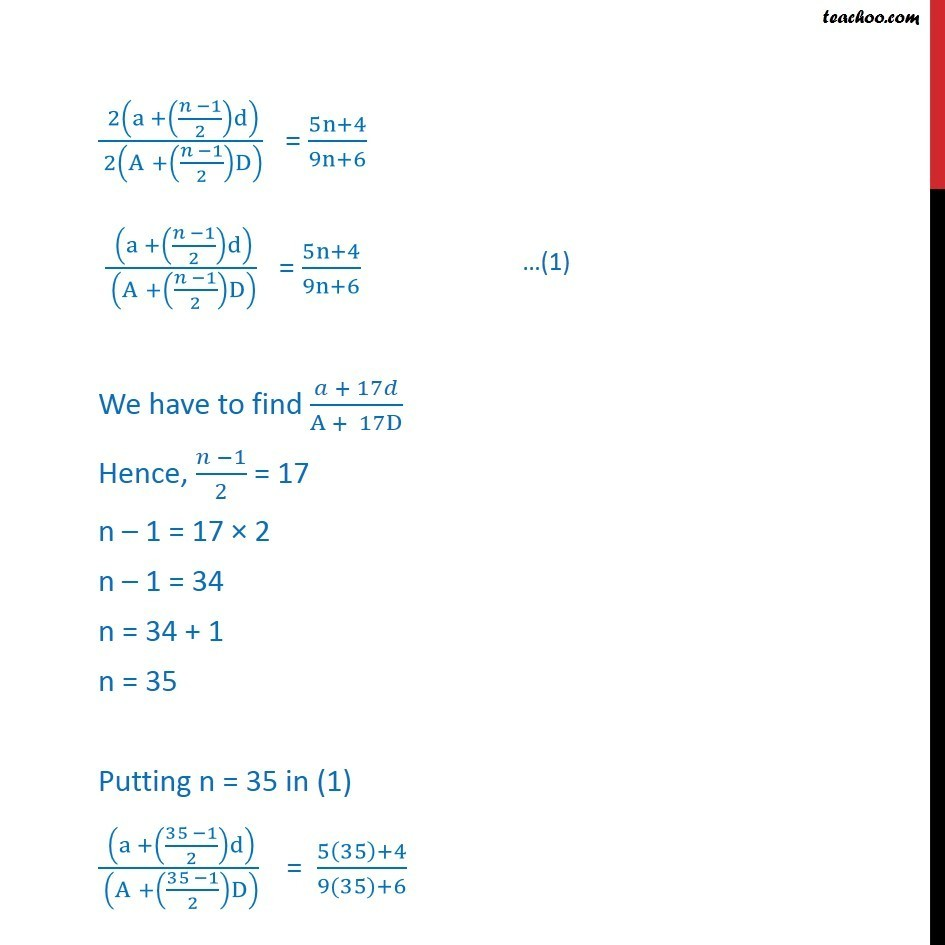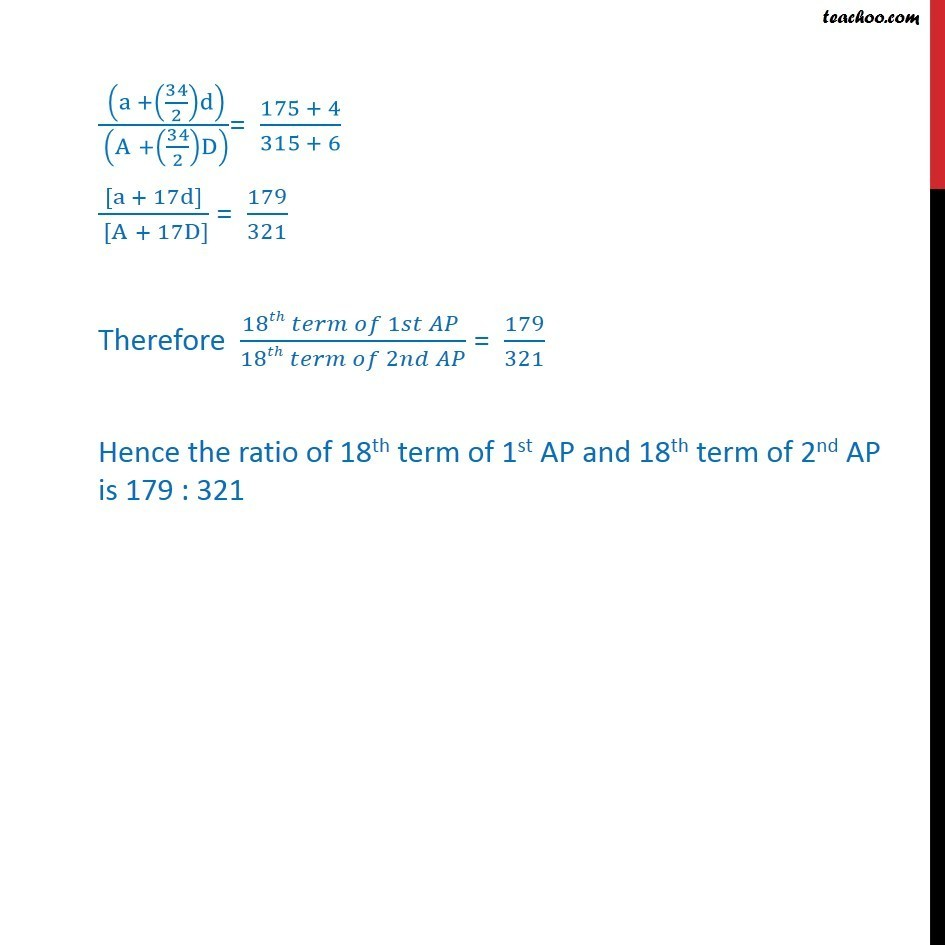Arithmetic Progression

Chapter 8 Class 11 Sequences and Series
Serial order wiseLearn in your speed, with individual attention - Teachoo Maths 1-on-1 Class

### Transcript

Ex 9.2 , 9 The sums of n terms of two arithmetic progressions are in the ratio 5n + 4: 9n + 6. Find the ratio of their 18th terms. There are two AP with different first term and common difference For the first AP Let first term be = a Common difference = d Sum of n terms = Sn = /2 [2a + (n 1)d] & nth term = an = a + (n 1)d For the second AP Let first term be = A common difference = D Sum of n terms = Sn = /2 [2A + (n 1)D] & nth term = An = A + (n 1)D We need to find ratio of their 18th term i.e. (18 1 )/(18 2 ) = ( 18 1 )/( 18 2 ) = (a + (18 1)d)/(A + (18 1)D) = ( + 17 )/(A + 17D) is given that (Sum of n terms of first A )/(Sum of n terms of second A ) = (5n+4)/(9n+6) ( /2[2 +( 1) ])/(( )/2[2 +( 1) ]) = (5n+4)/(9n+6) ( [2 +( 1) ])/( [2 +( 1) ]) = (5n+4)/(9n+6) ( 2(a +(( 1)/2)d))/( 2(A +(( 1)/2)D) ) = (5n+4)/(9n+6) ( (a +(( 1)/2)d))/( (A +(( 1)/2)D) ) = (5n+4)/(9n+6) We have to find ( + 17 )/(A + 17D) Hence, ( 1)/2 = 17 n 1 = 17 2 n 1 = 34 n = 34 + 1 n = 35 Putting n = 35 in (1) ( (a +((35 1)/2)d))/( (A +((35 1)/2)D) ) " "= (5(35)+4)/(9(35)+6) ( (a +(34/2)d))/( (A +(34/2)D) )= (175 + 4)/(315 + 6) ([a + 17d])/( [A + 17D]) = 179/321 Therefore (18 1 )/(18 2 ) = 179/321 Hence the ratio of 18th term of 1st AP and 18th term of 2nd AP is 179 : 321